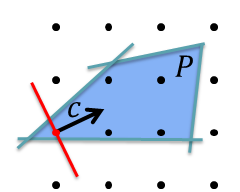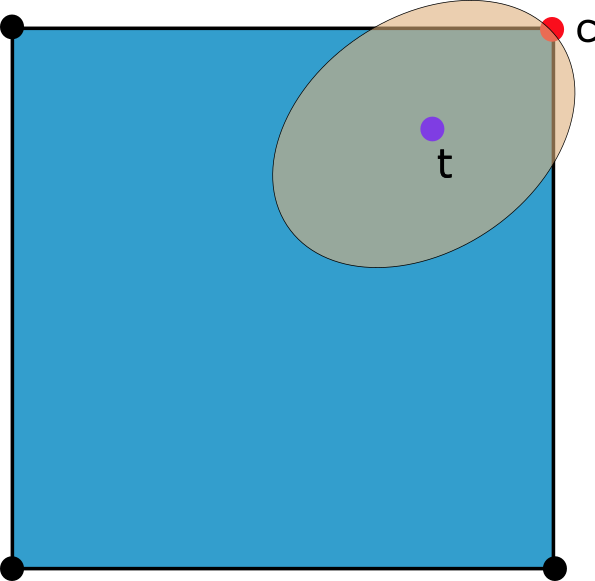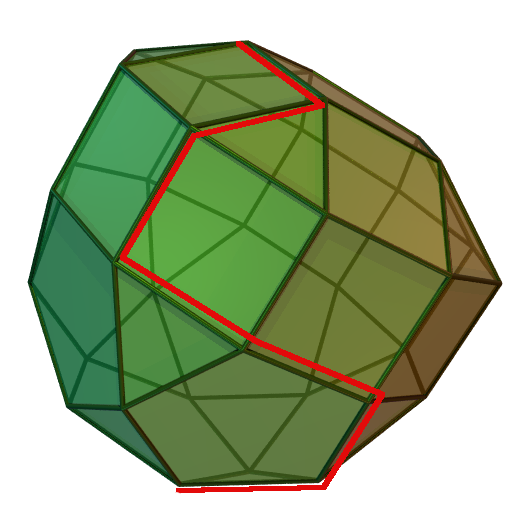## Towards a Quantitative Theory of Integer Programming (QIP)### Summary

Integer programming (IP), i.e. linear optimization with integrality constraints on variables, is one of the most successful methods for solving large scale optimization problems in practice. While many of the base IP problems such as the traveling salesman problem (TSP) or satisfiability (SAT) are NP-Complete, IPs with tens of thousands of variables are routinely solved in just a few hours by current state of the art IP solvers. The main goal of this proposal is to develop a quantitative theory capable of explaining when and how well different IP solver techniques will work on a wide range of instances. Here we will study many of the principal tools used to solve IPs including branch \& bound, the simplex method, cutting planes and rounding heuristics. Our first direction of study will be to develop parametrized classes of instances, inspired by the structure of realistic models, on which branch \& bound and the simplex method are provably efficient. The second research direction will be to develop alternatives to ad hoc rounding heuristics and cutting plane selection strategies with provable guarantees and provide their applications to important classes of IPs. Lastly, we will explore the power and limitations of IP techniques in the context of algorithm design by comparing them to powerful techniques in theoretical computer science and analyzing their worst-case performance for solving general integer programs. While the main thrust of this proposal is theoretical, it will be complimented by an experimental component performed in collaboration with well-known experts in computational IP, both to gain valuable insights on the structure of real-world instances and to validate the effectiveness newly suggested approaches. The proposed research is designed to make breakthroughs in our quantitative understanding of IP techniques, many of which have long resisted theoretical analysis.

### Project Publications:

1. Revisiting Tardos' Framework for Linear Programming: Faster Exact Solutions using Approximate Solvers.
D. Dadush, B. Natura, L. Végh. To appear in FOCS 2020.
2. On the Complexity of Branching Proofs
D. Dadush, S. Tiwari. CCC 2020 (Best paper award).
3. A Scaling-Invariant Algorithm for Linear Programming whose Running Time Depends only on the Constraint Matrix
D. Dadush, S. Huiberts, B. Natura, L. Végh. STOC 2020.

### Structure of Lattice PointsDescription: Ever since the 1980's, following the breakthrough works of Lenstra 82 and Kannan 87, the complexity to for solving an integer inequality systems $Ax \leq b, x \in \Z^n$ on n variables (IP) has been stuck at $n^{O(n)}$. The main bottleneck is a beautiful question in the geometry of numbers: what is the best way to decompose an almost integer point free n-dimensional convex body? A conjecture of Kannan & Lovasz 88 posits that there is an "easy way" to decompose any such body using $(\log n)^{O(k)}$ pieces of dimension $n-k$ for the right choice of $k$, which leaves open the possibility of a $(\log n)^{O(n)}$ algorithm for IP. This conjecture has very recently been verified for the important special case of ellipsoids together with an efficient algorithm for computing these decompositions.

Given the recent progress, the main thrust of this project will be to fully resolve the Kannan & Lovasz conjecture. This will involve developing new tools in convex geometry and the geometry of numbers.

### Discrepancy MinimizationDescription: A crucial task in many approximation algorithms is to round a fractional solution to a nearby zero-one solution. Discrepancy minimization is a powerful and flexible technique for performing this rounding while incurring "small error". Most generally, one considers the problem of given a norm (which quantifies the error), desired error $\epsilon$ and a point $t$ in the $[0,1]^n$ cube, to find a 0/1 point in the cube at distance $\epsilon$ from $t$ (assuming it exists). Techniques to solve this problem were recently applied to improve the additive approximation for the classical bin packing problem, and many more applications seem to be on the horizon.

An important research direction we will pursue, which was initiated by the breakthrough work of Bansal in 2010, is to design efficient algorithms for achieving discrepancy guarantees that were previously only known existentially. In particular, we will focus on making algorithmic a result of Banaszczyk for the so-called vector balancing problem, which has numerous applications. Improving on the above vein, we will find ways to make known discrepancy algorithms more efficient and flexible. In particular, it is often the case that one would like to be able to combine the discrepancy guarantees from different algorithms together, and understanding when this is possible will be an important focus of the research.

As a second major direction, there are many discrepancy questions where the best known existential bounds are believed to be far from tight. One such question is one of Steinitz, which asks when we can permute an input sequence of vectors such that its prefix sums have small norm. Both improved algorithms and bounds for this problem would have many interesting consequences.

### Linear ProgrammingDescription: Linear programming (LP), i.e. solving systems of the form $\max c \cdot x, Ax \leq b, x \in \R^n$, has a vast number of applications and represents one of the most fundamental classes of Optimization problems. To cope with the growing complexity of analyzing and planning based on a deluge of data, there is a growing need to be able to solve huge LPs that many current methodologies are unable to handle. On a more basic level, the factors determining how difficult LPs are to solve from a theoretical perspective are still poorly understood.

Over the last few years, tremendous theoretical progress has been made in the context of interior point methods (IPMs), which represent the current most theoretically efficient algorithms for LP. Most of the progress on interior point methods has been aimed at the complexity of approximately solving LPs, however many important applications require exact solutions. A main goal of the research here will to develop faster IPMS for exact LP solving.

As the second research vein, we will improve our understanding of the most popular method used in practice today: the simplex method developed by Dantzig 60 years ago. While not polynomial in general, the method is heavily used because of its good practical performance on "real life instances", and perhaps most importantly, because it is very well adapted for solving sequences of related linear programs (often arising from adding cuts to LP relaxations of integer programs). One major research push will be to improve known bounds for the running time of the simplex method on "realistic instances", in particular, using the smoothed analysis framework of Spielman and Teng. Secondly, we will attempt to give theoretical justification for why the simplex method performs well on related LPs, a subject which has received almost no theoretical attention.